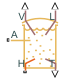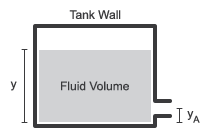# Tank (TL)

Thermal liquid container with variable fluid volume

•Libraries:
Simscape / Fluids / Thermal Liquid / Tanks & Accumulators

## Description

The Tank (TL) block models a thermal liquid container with a variable fluid volume. The absolute pressure of the tank fluid volume is assumed constant and equal to the value specified in the block dialog box. In the special case that the tank pressurization is equal to atmospheric pressure, the block represents a vented tank.

The tank can exchange energy with its surroundings, allowing its internal temperature and pressure to evolve over time. Heat transfer occurs via convection, as liquid enters or exits the chamber, and conduction, as thermal energy flows through the tank walls and the liquid itself at the tank inlets.

Tank SchematicThe tank can have up to six input ports, A through F. The pressure at the tank inlets is the sum of the constant tank pressurization specified in the block dialog box and the hydrostatic pressure due to the inlet height.

The tank model accounts for heat transfer through the tank wall, associated with thermal conserving port H. The temperature defined at this port is the temperature of the tank fluid volume.

### Tank Volume

The tank fluid volume is computed from the total fluid mass at each time step:

`$V=\frac{M}{\rho },$`

where:

• V is the tank fluid volume.

• M is the tank fluid mass.

• ρ is the tank fluid density.

### Mass Balance

The mass conservation equation in the tank fluid volume is

`$\stackrel{˙}{M}={\stackrel{˙}{m}}_{A}+{\stackrel{˙}{m}}_{B}+{\stackrel{˙}{m}}_{C}+{\stackrel{˙}{m}}_{D}+{\stackrel{˙}{m}}_{E}+{\stackrel{˙}{m}}_{F},$`

where:

• $\stackrel{˙}{M}$ is the change in fluid mass.

• ${\stackrel{˙}{m}}_{\eta }$ denotes the mass flow rates into the tank fluid volume through port η.

### Momentum Balance

The momentum conservation equation in the tank fluid volume for port η with $\eta \text{=A,B,C,D,E,F}$ is

`${p}_{\eta }+{p}_{dyn}={p}_{Ref}+\rho g\left(y-{y}_{\eta }\right),$`

where:

• pη is the fluid pressure at inlet η.

• pRef is the constant tank pressurization.

• pdyn is the dynamic pressure:

`${p}_{dyn}=\left\{\begin{array}{ll}0,\hfill & {\stackrel{˙}{m}}_{\eta }\ge 0\hfill \\ \frac{{\stackrel{˙}{m}}_{\eta }^{2}}{2{\rho }_{\eta }{S}_{IA}^{2}},\hfill & {\stackrel{˙}{m}}_{\eta }<0\hfill \end{array}$`

When the flow direction is into tank, the incoming jet dissipates into the large fluid volume, loses momentum, and causes pdyn to be `0`. When the fluid flows out of the tank, the fluid in the volume accelerates into the port and causes pdyn to be greater than `0`.

• ρη is the liquid density at port η.

• SIA is the tank inlet area.

• g is the gravitational constant.

• y is the tank level, or height, relative to the tank bottom.

• yη is the tank inlet elevation relative to the tank bottom.

### Energy Balance

The energy conservation equation in the tank fluid volume is

`$M\left({C}_{p}-h\alpha \right)\stackrel{˙}{T}={\varphi }_{A}+{\varphi }_{B}+{\varphi }_{C}+{\varphi }_{D}+{\varphi }_{E}+{\varphi }_{F}-\left({\stackrel{˙}{m}}_{A}+{\stackrel{˙}{m}}_{B}+{\stackrel{˙}{m}}_{C}+{\stackrel{˙}{m}}_{D}+{\stackrel{˙}{m}}_{E}+{\stackrel{˙}{m}}_{F}+M\alpha \stackrel{˙}{T}\right)h+Q,$`

where:

• Cp is the fluid thermal capacity.

• α is the fluid isobaric bulk modulus.

• T is the fluid temperature.

• Φη denotes the energy flow rates into the tank through port η.

• h is the fluid enthalpy.

• Q is the thermal energy flow rate into the tank through port H.

## Assumptions and Limitations

• The tank pressure is constant and uniform throughout the tank volume. The tank elevation head affects only the inlet pressure calculations.

• Fluid momentum is lost at the tank inlet due to the sudden expansion into the tank volume.

## Ports

### Conserving

expand all

Tank inlet.

Optional tank inlet.

#### Dependencies

To enable this port, set Number of inlets to `2`, `3`, `4`, `5`, or `6`.

Optional tank inlet.

#### Dependencies

To enable this port, set Number of inlets to `3`, `4`, `5`, or `6`.

Optional tank inlet.

#### Dependencies

To enable this port, set Number of inlets to `4`, `5`, or `6`.

Optional tank inlet.

#### Dependencies

To enable this port, set Number of inlets to `5`, or `6`.

Optional tank inlet.

#### Dependencies

To enable this port, set Number of inlets to `6`.

Heat transfer at the tank wall.

### Output

expand all

Liquid volume inside the tank in `m^3`, specified as a physical signal.

Liquid level inside the tank in `m`, specified as a physical signal.

Tank fluid temperature in `K`, specified as a physical signal.

## Parameters

expand all

Number of inlet ports. Setting this parameter to `2` or more exposes additional input ports.

Specifies a constant tank pressure. You can define a non-atmospheric pressure by selecting `Specified pressure` and defining the value in Tank pressurization.

User-defined tank pressure.

Specifies tank area characteristics. This parameter is used to determine the fluid level in the tank. If you would like to model a tank with a variable cross-sectional area, you can provide data for tank volume and fluid level in the ```Tabulated data - volume vs. level``` option.

Tank cross-sectional area.

#### Dependencies

To enable this parameter, set Tank volume parameterization to ```Constant cross-section area```.

Vector of tank fluid levels for the tabular parameterization of a non-constant tank area. The values in this vector correspond one-to-one to values in the Volumetric flow rate vector parameter. Its elements are listed in ascending order. The elements must be positive and the first element must be 0.

#### Dependencies

To enable this parameter, set Tank volume parameterization to ```Tabulated data - volume vs. level```.

Vector of tank fluid levels for the tabular parameterization of a non-constant tank area. The values in this vector correspond one-to-one to values in the Volumetric flow rate vector parameter. Its elements are listed in ascending order. The elements must be positive and the first element must be 0.

#### Dependencies

To enable this parameter, set Tank volume parameterization to ```Tabulated data - volume vs. level```.

Height of inlet port. The value must be greater than or equal to 0.

#### Dependencies

To enable this parameter, set Number of inlets to `1`.

Cross-sectional area of the port inlet. This value must be greater than 0.

Vector of port heights for multiple enabled ports. If you have two or more ports enabled, the Inlet height parameter becomes a vector of values that correspond to the height of each inlet port, starting with port A. The parameter name and vector length depend on the value of the Number of inlets parameter. The default height for each inlet is `.1 m`. Each element of this vector must be greater than or equal to 0.

#### Dependencies

To enable this parameter, set Number of inlets to `2`, `3`, `4`, `5`, or `6`.

Vector of cross-sectional areas for multiple enabled ports. If you have two or more ports enabled, the Inlet cross-sectional area parameter becomes a vector of values that correspond to the cross-sectional area of each inlet, starting with port A. The parameter name will include all enabled ports. Each element of this vector must be greater than 0.

#### Dependencies

To enable this parameter, set Number of inlets to `2`, `3`, `4`, `5`, or `6`.

Whether to be notified if the tank fluid level falls below the port inlet height during simulation. Set this parameter to `Warning` if you would like to receive a warning when this occurs during simulation. Set the parameter to `Error` if you would like the simulation to stop when this occurs.

Whether to be notified if the tank fluid volume rises above the tank maximum capacity during simulation. Set this parameter to `Warning` if you would like to receive a warning when this occurs during simulation. Set the parameter to `Error` if you would like the simulation to stop when this occurs.

Fill limit of the tank.

#### Dependencies

To enable this parameter, set Liquid volume above max capacity to either:

• `Warning`

• `Error`

Constant for the acceleration of gravity.

## Version History

Introduced in R2016a